# 廣義量詞系列：量詞的關係性質

## 2. 預備知識

### 2.1 集合與數字形式

every(A)(B) ⇔ A ⊆ B ⇔ A − B = Φ
every[x, y] ⇔ x = 0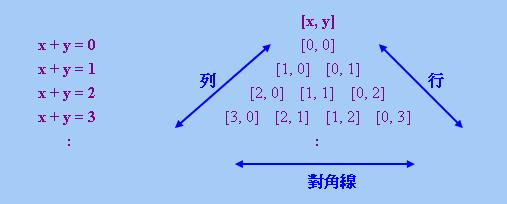「邏輯限定詞」的各種性質的定義也可以表現為集合和數字這兩種形式。Westerstahl指出，如果有關定義採取「純全稱量化」(即除了「全稱量詞」外，不含其他量詞)的形式，那麼有一種機械的方法把該定義從集合形式轉換為數字形式。假設有關定義的集合形式僅涉及兩個集合變項A和B，那麼我們可以利用x = |A − B|，y = |A ∩ B|，z = |B − A|和u = |U − (A ∪ B)|以及前述「集合論元」與「數字論元」的關係，把集合形式轉換為數字形式。舉例說，下文將會介紹的「連通性」的集合定義為

∀A∀B (Q(A)(B) ∨ Q(B)(A) ∨ A = B)

∀A∀B (Q[|A − B|, |A ∩ B|] ∨ Q[|B − A|, |B ∩ A|] ∨ (every(A)(B) ∧ every(B)(A)))

∀x∀y∀z (Q[x, y] ∨ Q[z, y] ∨ x = z = 0)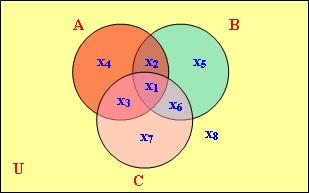∀A∀B∀C (Q(A)(B) ∧ Q(B)(C) ⇒ Q(A)(C))

∀x1∀x2∀x3∀x4∀x5∀x6 ((Q[x3 + x4, x1 + x2] ∧ Q[x2 + x5, x1 + x6]) ⇒ Q[x2 + x4, x1 + x3])

### 2.2 非平凡性與變異性

TRIV = {1, 0}       (1)

∀A∀ B (~Q(A)(B) ⇒ Q(A)(B))
∀A∀ B (Q(A)(B) ⇒ ~Q(A)(B))

VAR ⊆ ~TRIV       (4)

## 3. 各種關係性質的定義

### 3.1 基本定義

「自反性」(Reflexivity)
REF
Q(A)(A)
「否自反性」(Irreflexivity)
I-REF
~Q(A)(A)
「準自反性」(Quasireflexivity)
Q-REF
Q(A)(B) ⇒ Q(A)(A)
「弱自反性」(Weak Reflexivity)
W-REF
Q(A)(B) ⇒ Q(B)(B)
「準全集性」(Quasiuniversality)
Q-UNI
Q(A)(A) ⇒ Q(A)(B)
「弱全集性」(Weak Universality)
W-UNI
Q(B)(B) ⇒ Q(A)(B)
「強線性」(Strong Linearity)
S-LIN
Q(A)(B) ∨ Q(B)(A)
「對稱性」(Symmetry)
SYM
Q(A)(B) ⇒ Q(B)(A)
「否對稱性」(Asymmetry)
A-SYM
Q(A)(B) ⇒ ~Q(B)(A)
「反對稱性」(Antisymmetry)
AN-SYM
(Q(A)(B) ∧ Q(B)(A)) ⇒ A = B
「弱對稱性」(Weak Symmetry)
W-SYM
(Q(A)(B) ∧ Q(B)(C)) ⇒ Q(B)(A)
「連通性」(Connectedness)
CONN
Q(A)(B) ∨ Q(B)(A) ∨ A = B
「近連通性」(Almost-Connectedness)
AL-CONN
Q(A)(C) ⇒ (Q(A)(B) ∨ Q(B)(C))
「傳遞性」(Transitivity)
TRANS
(Q(A)(B) ∧ Q(B)(C)) ⇒ Q(A)(C)
「循環性」(Circularity)
CIRC
(Q(A)(B) ∧ Q(B)(C)) ⇒ Q(C)(A)
「歐幾里德性」(Euclidity)
EUCLID
(Q(A)(B) ∧ Q(A)(C)) ⇒ Q(B)(C)
「反歐幾里德性」(Antieuclidity)
AN-EUCLID
(Q(A)(B) ∧ Q(C)(B)) ⇒ Q(A)(C)

Q不是「自反」的當且僅當存在集合A，使得~Q(A)(A)
Q是「否自反」的當且僅當對所有集合A，均有~Q(A)(A)

Q ∈ P ⇔ ~Q ∈ P'

Q ∈ P ⇔ ~Q ∈ P

 定理1： (i) REF與I-REF互為「外補性質」 (ii) Q-REF與Q-UNI互為「外補性質」 (iii) W-REF與W-UNI互為「外補性質」 (iv) S-LIN與A-SYM互為「外補性質」 (v) AN-SYM與CONN互為「外補性質」 (vi) TRANS與AL-CONN互為「外補性質」 (vii) SYM為「自外補性質」

 (Q(A)(B) ∧ Q(B)(C)) ⇒ Q(A)(C) ≡ ~Q(A)(C) ⇒ ~(Q(A)(B) ∧ Q(B)(C)) ≡ ~Q(A)(C) ⇒ (~Q(A)(B) ∨ ~Q(B)(C))

### 3.2 六種性質的新定義

 定理2： 在LOG ∩ FIN下， (i) Q ∈ REF當且僅當A ⊆ B ⇒ Q(A)(B)，即x = 0 ⇒ Q[x, y] (ii) Q ∈ I-REF當且僅當Q(A)(B) ⇒ A ~⊆ B，即Q[x, y] ⇒ x ≠ 0 (iii) Q ∈ AN-SYM當且僅當Q(A)(B) ⇒ A ⊆ B，即Q[x, y] ⇒ x = 0 (iv) Q ∈ CONN當且僅當A ~⊆ B ⇒ Q(A)(B)，即x ≠ 0 ⇒ Q[x, y] (v) Q ∈ SYM當且僅當Q(A)(B) ⇔ Q(A ∩ B)(A ∩ B)，即Q[x, y] ⇔ Q[0, y] (vi) Q ∈ AN-EUCLID當且僅當Q(A)(B) ⇔ Q(A)(A)，即Q[x, y] ⇔ Q[0, x + y]

(Q[x, y] ∧ Q[z, y]) ⇒ x = z = 0       (5)

(Q[x3 + x4, x1 + x2] ∧ Q[x3 + x7, x1 + x6]) ⇒ Q[x2 + x4, x1 + x3]       (6)

Q[x, y] ⇒ Q[0, x + y]

Q[0, x + y] ⇒ Q[x, y]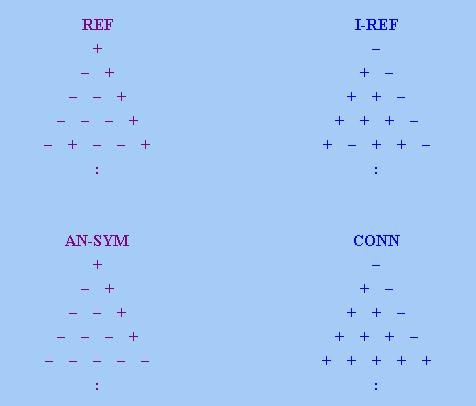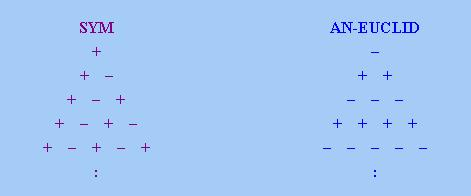### 3.3 傳遞性與近連通性

 定理3： 在LOG ∩ FIN下， (i) 若Q ∈ TRANS，則Q(A)(B) ⇒ (A ⊆ B ∨ Q(A)(Φ))，即Q[x, y] ⇒ (x = 0 ∨ Q[x + y, 0]) (ii) 若Q ∈ TRANS，則Q(A)(Φ) ⇒ Q(A)(B)，即Q[x + y, 0] ⇒ Q[x, y] (iii) 若Q ∈ TRANS，則(Q(A)(A) ∧ Q(C)(D) ∧ C ~⊆ D ∧ |C| > |A|) ⇒ Q(A)(B)， 即(Q[0, x + y] ∧ Q[v, w] ∧ v ≠ 0 ∧ v + w > x + y) ⇒ Q[x, y] (iv) Q ∈ TRANS當且僅當存在兩個由非負整數組成的集合S和T，使得S < T，並且 Q(A)(B) ⇔ |A| ∈ S ∨ (A ⊆ B ∧ |A| ∈ T)， 即Q[x, y] ⇔ x + y ∈ S ∨ (x = 0 ∧ x + y ∈ T) (v) Q ∈ AL-CONN當且僅當存在兩個由非負整數組成的集合S和T，使得S < T，並且 Q(A)(B) ⇔ |A| ∈ ~(S ∪ T) ∨ (A ~⊆ B ∧ |A| ∈ T)， 即Q[x, y] ⇔ x + y ∈ ~(S ∪ T) ∨ (x ≠ 0 ∧ x + y ∈ T)

(Q[x3 + x4, x1 + x2] ∧ Q[x2 + x5, x1 + x6]) ⇒ Q[x2 + x4, x1 + x3]       (7)

Q[x + 1, y + 1] ⇒ Q[x + 2, y]

(x, y > 0 ∧ Q[x, y]) ⇒ Q[x + 1, y − 1]

Q[x, y] ⇒ (x = 0 ∨ Q[x + y, 0])

(i)至此得證。

Q[x + y, 0] ⇒ Q[x, y]

(ii)乃得證。

(Q[0, x + y] ∧ Q[v', w']) ⇒ Q[x, y]

Q[0, y + 0] ∧ Q[z, 0] ∧ z ≠ 0 ∧ z + 0 > y + 0

Q(A)(B) ⇔ |A| ∈ S ∨ (A ⊆ B ∧ |A| ∈ T)

 ~(x + y ∈ S ∨ (x = 0 ∧ x + y ∈ T)) ⇔ x + y ~∈ S ∧ ~(x = 0 ∧ x + y ∈ T) ⇔ x + y ~∈ S ∧ (x ≠ 0 ∨ x + y ~∈ T) ⇔ x + y ~∈ S ∧ ((x ≠ 0 ∧ x + y ∈ T) ∨ x + y ~∈ T) ⇔ (x + y ~∈ S ∧ x ≠ 0 ∧ x + y ∈ T) ∨ (x + y ~∈ S ∧ x + y ~∈ T) ⇔ (x + y ∈ T ∧ x ≠ 0) ∨ x + y ∈ ~(S ∪ T)

(v)乃得證。

Q(A)(B) ⇔ |A| = 0 ∨ (A ⊆ B ∧ |A| ≥ 1)
Q'(A)(B) ⇔ A ~⊆ B ∧ |A| ≥ 1

Q和Q'分別是"every"和"(not every)"，容易驗證前者滿足「傳遞性」，後者滿足「近連通性」。其次，把S = {3}，T = {5, 6, ...}代入(iv)和(v)中的條件，所得限定詞分別為

Q''(A)(B) ⇔ |A| = 3 ∨ (A ⊆ B ∧ |A| ≥ 5)
Q'''(A)(B) ⇔ (|A| ∈ {0, 1, 2, 4}) ∨ (A ~⊆ B ∧ |A| ≥ 5)

(some or none of s1 or ... or sm; or all of t1 or ... or tn)

(some or none of s1 or ... or sm; or not all of t1 or ... or tn)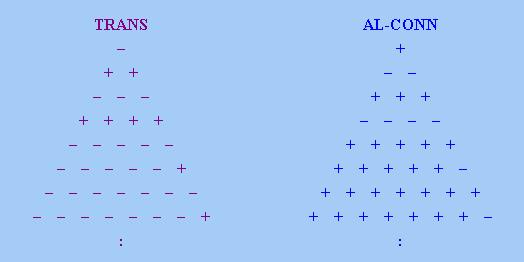## 4. 與關係性質有關的定理

### 4.1 某些性質之間的包含關係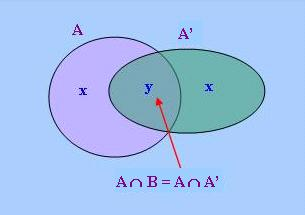定理5： 在LOG ∩ FIN下， (i) REF ⊆ W-REF ⊆ Q-REF (ii) I-REF ⊆ W-UNI ⊆ Q-UNI (iii) AN-SYM ⊆ TRANS ⊆ Q-REF (iv) CONN ⊆ AL-CONN ⊆ Q-UNI (v) SYM ⊆ W-SYM

(i)和(ii)的後半部可以從數字三角形的角度推得。根據表1，W-REF、Q-REF、W-UNI和Q-UNI的數字定義分別為

Q[x, y] ⇒ Q[0, y + z]       (8)
Q[x, y] ⇒ Q[0, x + y]       (9)
Q[0, y + z] ⇒ Q[x, y]       (10)
Q[0, x + y] ⇒ Q[x, y]       (11)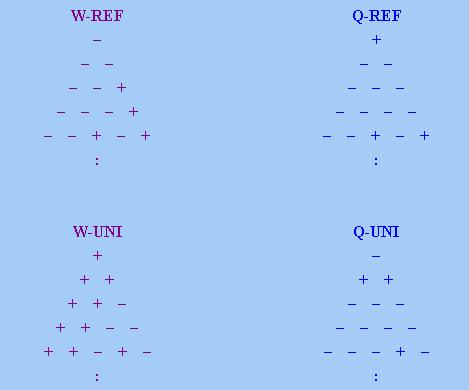### 4.2 一些否定性結果

 定理6： 在LOG ∩ FIN − TRIV下， (i) S-LIN = Φ (ii) A-SYM = Φ (iii) EUCLID = Φ (iv) CIRC = Φ

Q[x, y] ∨ Q[z, y]       (12)

(Q[x3 + x4, x1 + x2] ∧ Q[x2 + x4, x1 + x3]) ⇒ Q[x2 + x5, x1 + x6]       (13)

Q[x, y] ⇒ Q[0, y + z]       (14)

Q[0, u] ⇒ Q[u, u]       (15)

Q[u, u] ⇒ Q[u, 0]       (16)

Q[u, 0] ⇒ Q[0, 0]       (17)

Q[0, 0] ⇒ Q[v, w]       (18)

 定理7： 在LOG ∩ FIN − TRIV下， (i) REF ∩ AL-CONN = Φ (ii) I-REF ∩ TRANS = Φ (iii) REF ∩ CONN = Φ (iv) I-REF ∩ AN-SYM = Φ (v) W-REF ∩ CONN = Φ (vi) W-UNI ∩ AN-SYM = Φ (vii) SYM ∩ TRANS = Φ (viii) SYM ∩ AL-CONN = Φ (ix) SYM ∩ REF = Φ (x) SYM ∩ I-REF = Φ (xi) REF ∩ I-REF = Φ (xii) AN-SYM ∩ CONN = Φ

S-LIN = REF ∩ CONN       (19)
A-SYM = I-REF ∩ AN-SYM       (20)

(20)可以證明如下：

 Q ∈ A-SYM ⇔ ~Q ∈ S-LIN (根據「定理1(iv)」) ⇔ ~Q ∈ REF ∩ CONN (根據(19)) ⇔ Q ∈ I-REF ∩ AN-SYM (根據「定理1(i)和(v)」)

(xi)可以根據REF和I-REF的集合定義直接推得，由於REF和I-REF的集合定義不可能同真，所以不可能有Q ∈ REF ∩ I-REF。

 定理8： 在LOG ∩ FIN ∩ VAR下， (i) AN-EUCLID = Φ (ii) Q-REF ∩ Q-UNI = Φ

AN-EUCLID = Q-REF ∩ Q-UNI

### 4.3 某些限定詞的特徵性質

 定理9： 在LOG ∩ FIN − TRIV下， (i) REF ∩ AN-SYM = {every} (ii) I-REF ∩ CONN = {(not every)} (iii) REF ∩ TRANS = ∪n ≥ 0{(some or none of n or fewer; or all of n + 1 or more)} (iv) I-REF ∩ AL-CONN = ∪n ≥ 0{(not all of n or more)} (v) W-REF ∩ SYM = {some, (at least 2), (at least 3) ...} (vi) W-UNI ∩ SYM = {no, (at most 1), (at most 2) ...} (vii) W-SYM ∩ AN-SYM = ∪n ≥ 0{(all of n)} (viii) W-SYM ∩ CONN = {(some or none of 1 or more)}

(Q[x, y] ∧ Q[y + z − w, w]) ⇒ Q[z, y]       (21)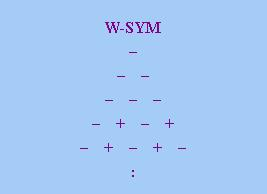定理10： 在LOG ∩ FIN ∩ VAR下， (i) AN-SYM = TRANS (ii) CONN = AL-CONN

 定理11： 在LOG ∩ FIN ∩ VAR下， (i) REF ∩ TRANS = {every} (ii) I-REF ∩ AL-CONN = {(not every)} (iii) W-REF ∩ SYM = {some} (iv) W-UNI ∩ SYM = {no} (v) W-SYM ∩ AN-SYM = Φ (vi) W-SYM ∩ CONN = Φ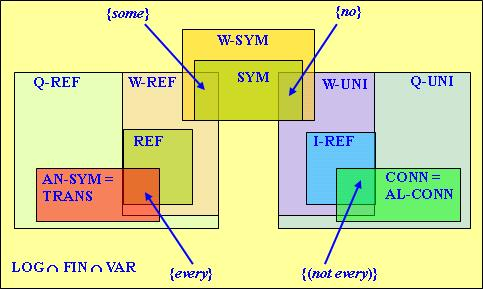### 4.4 與單調性的關係

 定理12： 在CONSr下， (i) REF ∩ TRANS ⊆ ↓MON↑ (ii) I-REF ∩ AL-CONN ⊆ ↑MON↓

「對稱性」與「單調性」有一種特殊的聯繫，詳見以下定理：

 定理13： 設Q ∈ SYM， (i) Q ∈ ↑MON ⇔ Q ∈ MON↑ (ii) Q ∈ ↓MON ⇔ Q ∈ MON↓

 定理14： 在CONSr ∩ SYM下， (i) Q-REF = ↑MON↑ (ii) Q-UNI = ↓MON↓

(ii)可以證明如下：

 Q ∈ Q-UNI ⇔ ~Q ∈ Q-REF (根據「定理1(ii)」) ⇔ ~Q ∈ ↑MON↑ (根據(i)) ⇔ Q ∈ ↓MON↓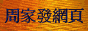<script type = "text/javascript"> (function(d, w) { var x = d.getElementsByTagName('SCRIPT'); var f = function() { var _id = 'lexity-pixel'; var _s = d.createElement('script'); _s.id = _id; _s.type = 'text/javascript'; _s.async = true; _s.src = "//np.lexity.com/embed/YW/be0aa169de7f441c6473361be62c9ef6?id=ddad453e7753"; if (!document.getElementById(_id)) { x.parentNode.insertBefore(_s, x); } }; w.attachEvent ? w.attachEvent('onload', f) : w.addEventListener('load', f, false); }(document, window)); </script>